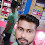### Program 91:Search a substring in a given string

Program 91:Search a substring in a given string

```#include<stdio.h>
#include<string.h>
main()
{
int i,j,flag=0,len1,len2;
char str,substr;
printf("Enter a string\n");
gets(str);
printf("Enter a substring to be searched\n");
gets(substr);
len1=strlen(str);
len2=strlen(substr);

for(i=0;i<=len1-len2;i++)
{
for(j=i;j<i+len2;j++)
{
flag=1;
if(str[j]!=substr[j-i])
{
flag=0;
break;
}
}
if(flag==1)
break;
}
if(flag==1)
{
printf("Search is Successful\n");
}
else
{
printf("Search is not Successful\n");
}
}```
Explanation:

1. This program starts with initializing :
• i,j → used as helping variable
• str → To store input from user
• substr To store substring to search
• len1,len2→ To store length of str and sustr
• flag →To use this for checking whether the given substr is present in str
2. ```printf("Enter a string\n");
gets(str);
printf("Enter a substring to be searched\n");
gets(substr);```
Taking input from user where str represents string(let's take "all c program") and substr represents substring("lets take "all c" as substring)
3. ```for(i=0;i<=len1-len2;i++)
{```
length of "all c programs" is 14(i.e from 0 to 13) in arrays and "all c" is 5. So len1=14 and len2=5.len1-len2=9.The loop iterates 10 times from 0 to 9.
4. Why len1-len2?
` `
As substring length is 5 then, if the loop traverses till the last but 5 letters without output i.e from 0 to 9 then remaining letters, after the 9th letter there are 4(all c programs having length of  14 i.e from 0-13 in arrays) letters which will not have enough letters for searching the substring.
5. Main logic
```for(j=i;j<i+len2;j++)
{
flag=1;
if(str[j]!=substr[j-i])
{
flag=0;
break;
}
}//end of forloop
if(flag==1)
break;```
i will traverse from 0 to 9(len1-len2) for each iteration i will be incremented by 1.len1=14,len2=5
• Iteration 1:j=i →j=0,j<0+5 which is true so loop is executed
•  j=0
• flag=1 by default when ever the loop is entered
• now str!=substr[0-0]→a!=a which is false so flag remains same
• now j is incremented by 1 so j=1
• Iteration 2:j=1 ,1<0+5 which is true so loop is executed
•  j=1,flag=1
• now str!=substr[1-0]→l!=l which is false so flag remains same
•  now j is incremented by 1 so j=2
• Iteration 3:j=2 ,2<0+5 which is true so loop is executed
•  j=2,flag=1
• now str!=substr[2-0]→l!=l which is false so flag remains same
•  now j is incremented by 1 so j=3
• Iteration 4:j=3 ,3<0+5 which is true so loop is executed
•  j=3,flag=1
• now str!=substr[3-0]→space!=space which is false so flag remains same
•  now j is incremented by 1 so j=4
• Iteration 5:j=4 ,4<0+5 which is true so loop is executed
•  j=4,flag=1
• now str!=substr[4-0]→c!=c which is false so flag remains same
•  now j is incremented by 1 so j=5
• Iteration 4:j=5,5<0+5 which is false so loop is terminated
• So the if part is never executed at all in 5 iterations so flag is remained as 1
• finally when flag=1 then the main loop for(i=0;i<len1-len2;i++) is terminated as if(flag==1){break;} is there inside the 1st forloop
6. ```if(flag==1)
{
printf("Search is Successful\n");
}
else
{
printf("Search is not Successful\n");
}```
As flag ==1 which is true so the if part is executed So Search is successful

Output:

#### 1 comment:

1.college-party-guide One of the most important rituals for students in college is to throw the most memorable student dorm celebration.
factorial hundred In the last few days, the “factorial of 100” is one of the top subjects and a lot of maths geeks compute it using voice assistants such as Alexa, Shiri, etc.
factorial hundred In the last few days, the “factorial of 100” is one of the top subjects and a lot of maths geeks compute it using voice assistants such as Alexa, Shiri, etc.
gigolo The term”gigolo” usually refers to the person who lives an arranged lifestyle that includes many of these relationships in a series, rather than having any other support system.